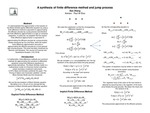## Stander Symposium Projects

Dan Zhang

#### Description

It is demonstrated that approximation of the solution of the Black-Scholes partial differential equation by using a finite difference method is equivalent to approximating the diffusion process by a jump process and therefore the finite difference approximation is a type of numerical integration. In particular, we establish that the explicit finite difference approximation is equivalent to approximating to diffusion process by a jump process, initially introduced by Cox and Ross, while the implicit finite difference approximation amounts to approximating the diffusion process by a more general type of jump process. This work has been introduced by Brennan and Schwartz, The Journal of Financial and Quantitative Analysis,  (1978).

4-18-2012

Paul W. Eloe

Mathematics

#### Keywords

Stander Symposium posterCOinS